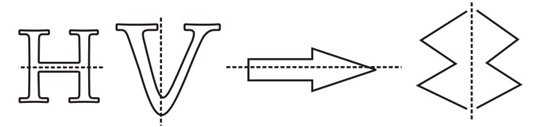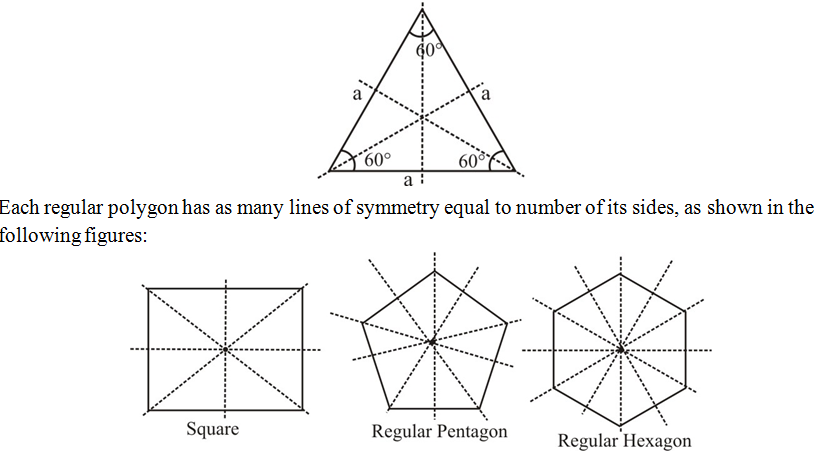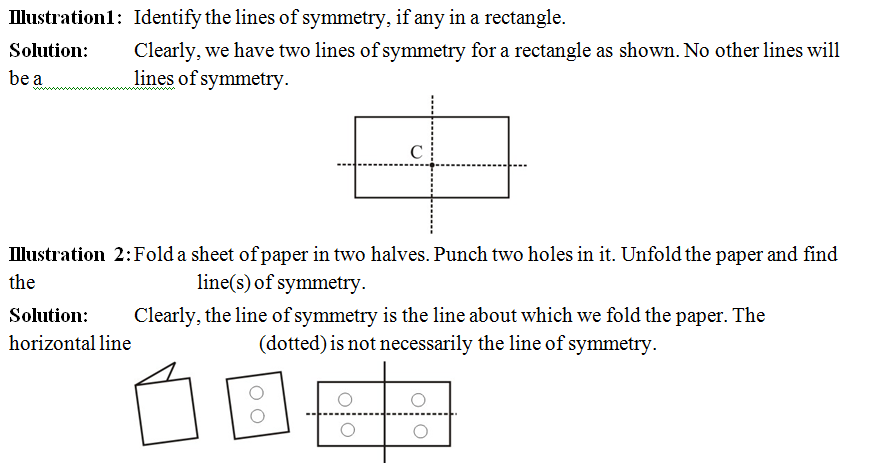# Introduction

## Introduction

Symmetry is a very important geometrical concept used in almost every field. You may be already familiar with line symmetry.The concept of line symmetry is closely related to mirror reflection. In the following figures the lines of symmetry (or mirror line) are marked with dotted lines.### Lines of Symmetry For Regular Polygons

A polygon is called regular if all its sides are equal in length and all its angles are also equal. An equilateral triangle is a regular polygon with the least number of the lines segments. The dotted lines show the symmetry. It has three lines of symmetry.